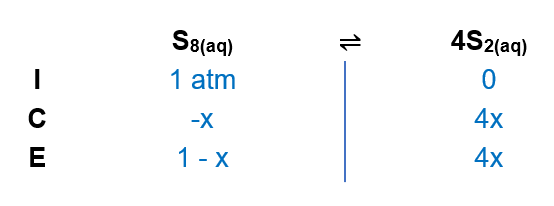# Problem: A sample of S8(g) is placed in an otherwise empty rigid container at 1325 K at an initial pressure of 1.00 atm, where it decomposes to S2(g) by the reactionS8(g) ⇌ 4S2(g)At equilibrium, the partial pressure of S8 is 0.25 atm. Calculate Kp for this reaction at 1325 K.

###### FREE Expert Solution

(1) Setup an ICE chart(2) At equilibrium, S8 temperature is 0.25 which means:

1 - x = 0.25

x = 0.75

98% (341 ratings)###### Problem Details

A sample of S8(g) is placed in an otherwise empty rigid container at 1325 K at an initial pressure of 1.00 atm, where it decomposes to S2(g) by the reaction

S8(g) ⇌ 4S2(g)

At equilibrium, the partial pressure of S8 is 0.25 atm. Calculate Kp for this reaction at 1325 K.

Frequently Asked Questions

What scientific concept do you need to know in order to solve this problem?

Our tutors have indicated that to solve this problem you will need to apply the ICE Chart concept. You can view video lessons to learn ICE Chart. Or if you need more ICE Chart practice, you can also practice ICE Chart practice problems.

What professor is this problem relevant for?

Based on our data, we think this problem is relevant for Professor Al-Nidawy & Green's class at University of Windsor.

What textbook is this problem found in?

Our data indicates that this problem or a close variation was asked in Chemistry: An Atoms First Approach - Zumdahl Atoms 1st 2nd Edition. You can also practice Chemistry: An Atoms First Approach - Zumdahl Atoms 1st 2nd Edition practice problems.